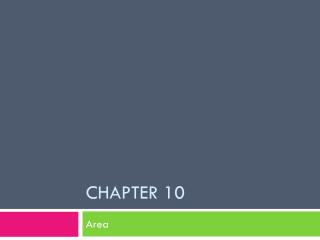DownloadDownload PresentationChapter 10

# Chapter 10

Download Presentation## Chapter 10

- - - - - - - - - - - - - - - - - - - - - - - - - - - E N D - - - - - - - - - - - - - - - - - - - - - - - - - - -
##### Presentation Transcript

1. Chapter 10 Area

2. Area of Rectangles and Parallelograms Area = bh Where b is the base and h is the height perpendicular to the base A.k.a. altitude

3. Area of a triangle A = ½ bh

4. Examples. Find the area of the following to the nearest tenth. 1) 2) 3) 4)

5. Area of a trapezoid

6. Example:

7. Area of a Kite and Rhombus

8. Examples: Find the area to the nearest tenth. 1) 2)

9. Day 2 Area of Polygons

10. Radius: From the center of the polygon to a vertex of the polygon. Used to find the apothem

11. Areas of Regular Polygons

12. Areas of Regular Polygons Perimeter Apothem

13. Theorem 10-6: Area of a Regular Polygon The area of a regular polygon is half the product of the apothem and the perimeter. A= ½ ap a = apothem p = perimeter

14. Example 1 Find the area of the octagon 9 in 7 in

15. Examples • Find the area of each of the following: 11 yd 9 yd 12 cm 4 in 11 cm 9 in

16. Using Special Right triangles • Use the properties of special right triangles to find the area of the hexagon. 10 in

17. More Examples • Using properties of special right triangles, find the area of each hexagon. 6 3 m 12 yd

18. Trigonometry and Area Find the area of the regular pentagon with 8cm sides. A = ½ (a)(40) 8 cm To find a: • Find the measure of < XCZ: • 360/5 = 72 C • Find the measure of < XCY: • 72/2 = 36 36 3. Use the triangle and trig to solve for a: a o = tan 36 = 4 a a a = 4 tan 36 a = 5.51 Use a to solve for area: A = ½ (5.51)(40) X 4 cm Z Y Area: 110.2 cm2

19. Find the area of a regular octagon with a perimeter of 80 in. Give the area to the nearest tenth. To find the angle measure: 360/8 = 45 45/2 = 22.5 10 in o = tan 22.5 = 5 a a a = 5 tan 22.5 a = 12.07 a Use formula: A = ½ (12.07)(80) 5 in A = 482.8 in2

20. Theorem 10.8: • The area of a triangle is one half the product of the lengths of two sides and the sine of the included angle. 3 Options:

21. Finding the Area of a Triangle Area of Triangle ABC = ½ bh Area = ½ bc(sin A) Area = ½ (52)(24)(sin 76) Area = 605.46 in2 B a 24 in h 76 A C 52 in

22. The surveyed lengths of two adjacent sides of a triangular plot of land are 412 ft and 386 ft. The angle between the sides is 71. Find the area of the plot. A = ½ bc(sin A) A = ½ (412)(386)(sin 71) 412 ft A = 75183.86 ft2 71 386 ft

23. Two sides of a triangular building plot are 120 ft and 85ft long. They include an angle of 85. Find the area of the building plot to the nearest square foot. A = ½ bc(sin A) 85 85 ft A = ½ (120)(85)(sin 85) 120 ft A = 5081 ft2

24. Warm Up • Find the area of a regular triangle with an apothem of 4”. • Find the area of a decagon with side lengths of 15 cm.

25. Using Trigonometry

26. Example

27. Circles and Arcs Radius Diameter Center

28. Central Angle Q R S An angle whose vertex is the center of the circle.

29. Arcs • Semicircle: half of the circle • Minor Arc: smaller than a semicircle • Major Arc: Greater than a semicircle

30. Example • Name three minor arcs, two semicircles, and three major arcs.

31. Adjacent Arcs • Arcs of the same circle that have exactly one point in common • Arc Addition Postulate: The measure of the arc formed by two adjacent arcs is the sum of the measures of the two arcs

32. Example • Find the measure of the following arcs 1. 2. 3. 4.

33. Concentric Circles • Circles in the same plane with the same center Not Concentric Concentric

34. Circumference In terms of pi Nearest tenth

35. Arc Length • The length of the fraction of the circle’s circumference

36. Area In terms of pi Nearest tenth

37. Sector • A region bounded by an arc of the circle and two radii

38. Area of a Sector • The product of the ratio of the sector and the area

39. Examples: Find the area of the sector shaded.

40. Segment • A part of a circle bounded by an arc and the segment joining its endpoints

41. Area of the segment:

42. Examples:

43. Find the area of the shaded region:

44. Geometric Probability

45. Find the probability of getting a dart in the center of this triangular board:

46. Find the probability of hitting one of the circles in the box with a dart:

47. 12-1 Tangent Lines

48. 12-1: Tangent Lines • Tangent to a circle: a line in the plane of the circle that intersects the circle in exactly one point. Point of Tangency